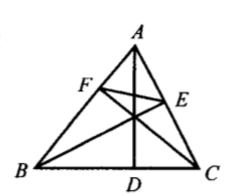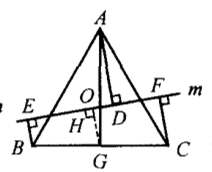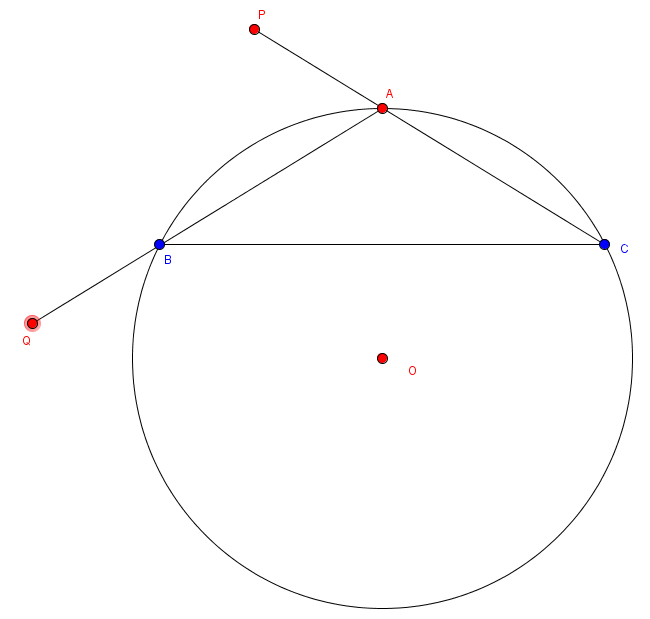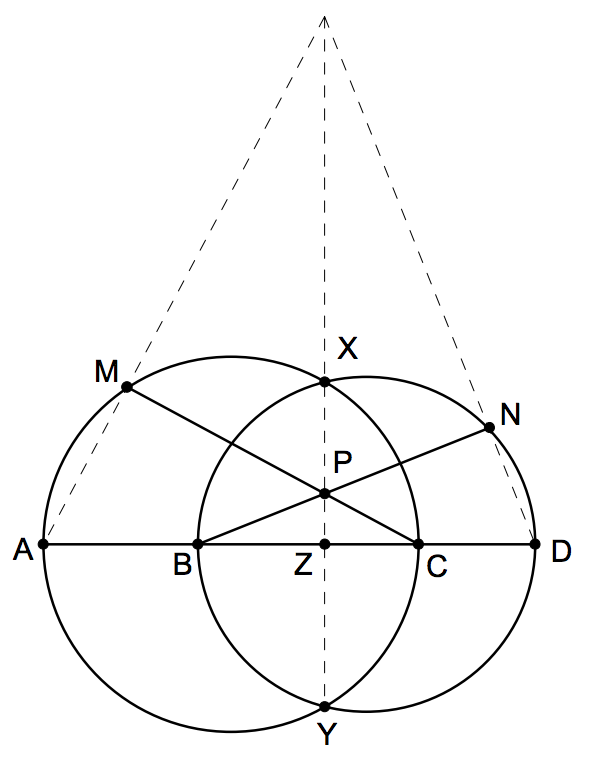###### back to index | new

Triangle $ABC$ has positive integer side lengths with $AB=AC$. Let $I$ be the intersection of the bisectors of $\angle B$ and $\angle C$. Suppose $BI=8$. Find the smallest possible perimeter of $\triangle ABC$.

Show that the three medians of a triangle intersect at one point.

In $\triangle{ABC}$, let $AD$, $BE$, and $CF$ be the three altitudes as shown. If $AB=6$, $BC=5$, and $EF=3$, what is the length of $BE$?Let $\triangle{ABC}$ be an acute triangle. If the distance between the vertex $A$ and the orthocenter $H$ is equal to the radius of its circumcircle, find the measurement of $\angle{A}$.

Let $AD$ be the altitude in $\triangle{ABC}$ from the vertex $A$. If $\angle{A}=45^\circ$, $BD=3$, $DC=2$, find the area of $\triangle{ABC}$.

Let $O$ be the centroid of $\triangle{ABC}$. Line $\mathcal{l}$ passes $O$ and intersects $AB$ and $AC$ at $P$ and $Q$, respectively. Point $D$, $E$, and $F$ are on the line $l$ such that $AD\perp l$, $BE \perp l$, and $CF\perp l$. Show that $AD = BE+CF$.Let $O$ be the incenter of $\triangle{ABC}$. Connect $AO$, $BO$, and $CO$ and extends so that they intersect with $\triangle{ABC}$'s circumcircle at $D$, $E$, and $F$, respectively. Let $DE$ intersect $AC$ at $G$, and $DF$ intersects $AB$ at $H$. Show that $G$, $H$ and $O$ are collinear.

Let $ABC$ be an acute triangle. Circle $O$ passes its vertex $B$ and $C$, and intersects $AB$ and $AC$ at $D$ and $E$, respectively. If $O$'s radius equals the radius of $\triangle{ADE}$'s circumcircle, then the circle $O$ must passes $\triangle{ABC}$'s (A) incenter (B) circumcenter (C) centroid (D) orthocenter.

Let $K$ is an arbitrary point inside $\triangle{ABC}$, and $D$, $E$, and $F$ be the centroids of $\triangle{ABK}$, $\triangle{BCK}$ and $\triangle{CAK}$, respectively. Find the value of $S_{\triangle{ABC}} : S_{\triangle{DEF}}$.

Let $I$ be the incenter of $\triangle{ABC}$. $AI$, $BI$, and $CI$ intersect $\triangle{ABC}$'s circumcircle at $D$, $E$, and $F$, respectively. Show that $EF \perp AD$

In $\triangle{ABC}$, $AB=AC$. Extending $CA$ to an arbitrary point $P$. Extending $AB$ to point $Q$ such that $AP=BQ$. Let $O$ be the circumcenter of $\triangle{ABC}$. Show that $A$, $P$, $Q$, and $O$ concyclic.In triangle ABC, M is median of BC. O is incenter. AH is altitude. MO and AH intersect at E. Prove that AE equal to the radius of incircle

Let $G$ be the centroid of $\triangle{ABC}$, $L$ be a straight line. Prove that $$GG'=\frac{AA'+BB'+CC'}{3}$$ where $A'$, $B'$, $C'$ and $G'$ are the feet of perpendicular lines from $A$, $B$, $C$, and $G$ to $L$.

Let $A$, $B$, $C$ and $D$ be four distinct points on a line, in that order. The circles with diameters $AC$ and $BD$ intersect at the points $X$ and $Y$. The line $XY$ meets $BC$ at the point $Z$. Let $P$ be a point on the line $XY$ different from $Z$. The line $CP$ intersects the circle with diameter $AC$ at the points $C$ and $M$, and the line $BP$ intersects the circle with diameter $BD$ at the points $B$ and $N$. Prove that the lines $AM$, $DN$ and $XY$ are concurrent(Euler Line) For any triangle $ABC$, show that the cicumcenter $O$, centroid $G$, and the orthocenter $H$ are collinear. Moreover, we have $OG:GH=1:2$.

(Euler Theorem) Let $ABC$ be a triangle, $O$, $I$ be respectively the circumcetner and incenter. Then $$OI^2 = R^2 - 2Rr$$, where $R$ denotes the circumradius and $r$ denotes the inradius.

(Nine-point circle) For any triangle $AB$C, the feet of three altitudes, the midpoints of three sides, and the midpoints of the segments from the vertices to the orthocenter, all lie on the same circle. This circle is of radius half as the circumradius of triangle $ABC$.

Show that the nine-point center $N$ lies on the Euler line, and is the middle point of $O$, the circumcenter, and $H$, the orthocenter.

Show that the triangles $ABC$, $ABH$, $BCH$, and $CAH$ all have the same nine-point circle, providing that the orthocenter $H$ does not coincide with each of the vertices $A$, $B$, $C$.

Let $a, b, c$ be respectively the lengths of three sides of a triangle, and $R$ be the triangle's circumradius. Show that $$R = \frac{abc}{\sqrt{(a+b+c)(b+c-a)(c+a-b)(b+a-c)}}$$

Prove the median's length formula: $$m_a = \frac{1}{2}\sqrt{2b^2 + 2c^2 - a^2}$$

Prove the triangle's altitude formula: $$h_a = \frac{2}{a}\sqrt{p(p-a)(p-b)(p-c)}$$

Prove the triangle's angle bisector length formula: $$t_a=\frac{2}{b+c}\sqrt{bcp(p-a)}=\sqrt{bc\Big(1-(\frac{a}{b+c})\Big)^2}$$

Let $\triangle{ABC}$ be a Pythagorean triangle. If $\triangle{ABC}$'s circumstance is 30, find its circumcircle's area.

Let $\triangle{ABC}$ be a right triangle whose sides lengths are all integers. If $\triangle{ABC}$'s perimeter is 30, find its incircle's area.